# Compare and order decimals#### All in One Place

Everything you need for better grades in university, high school and elementary.#### Learn with Ease

Made in Canada with help for all provincial curriculums, so you can study in confidence.#### Instant and Unlimited Help

0/2
##### Intros
###### Lessons
1. Introduction to Comparing and Ordering Decimals:
2. How to compare two decimal numbers
3. How to order a list of decimal numbers
0/15
##### Examples
###### Lessons
1. Comparing decimals using base ten (block) models
Write the decimal value represented by each base ten (block) model. Circle the greater value.
1.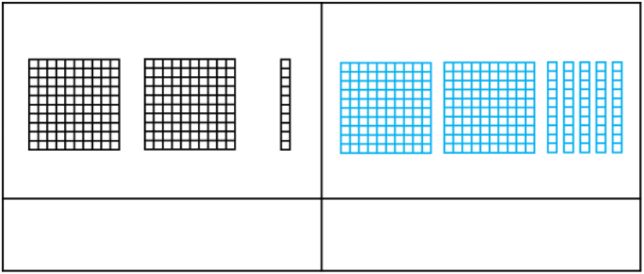2.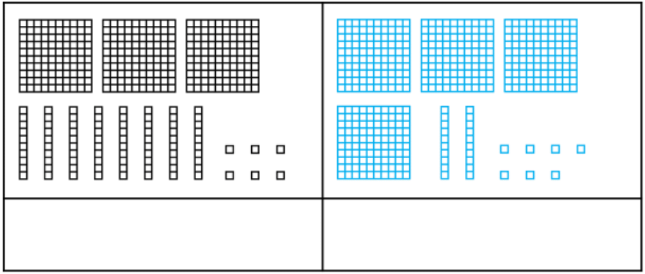3.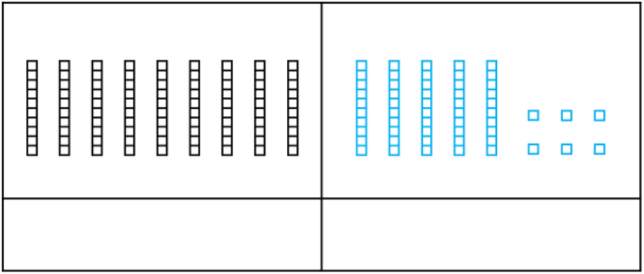2. Comparing decimals using place value digits
Fill out the value of digits for each decimal. Circle the lesser value.
1.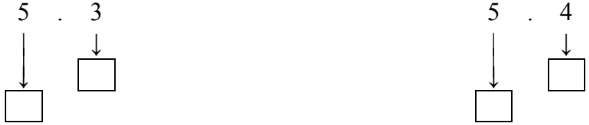2.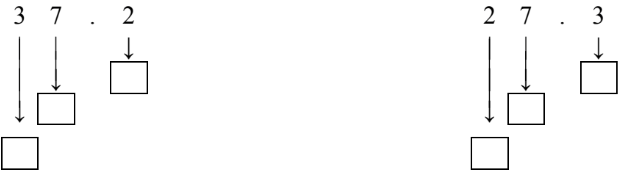3.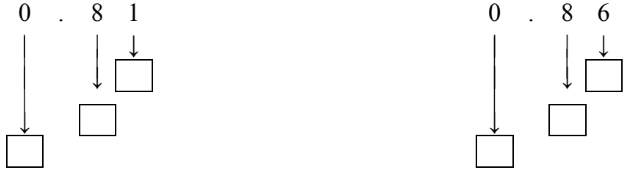4.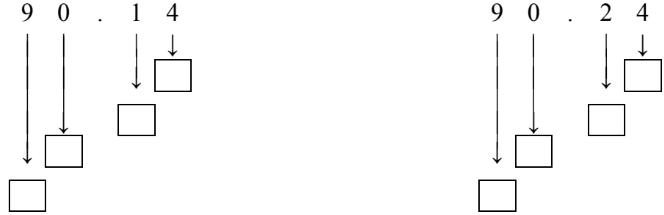3. Comparing decimals in a list
Compare the decimal numbers in the list and answer.
1. Which number is the biggest?
6.05, 50.6, 60.5, 56.0
2. Which number is the biggest?
8.72, 7.28, 8.27, 7.82
3. Which number is the smallest?
34.9, 39.4, 9.34, 4.93
4. Which number is the smallest?
10.62, 6.12, 16.02, 6.21
4. Ordering decimals
Order the decimals in the specified order using > or < symbols
1. Descending order (greatest to least)
1.2, 1.5, 1.0, 1.1
2. Ascending order (least to greatest)
4.83, 4.75, 4.82, 4.79
3. Descending order (greatest to least)
6.1, 6.9, 6.95, 6.02
4. Ascending order (least to greatest)
5.09, 3.71, 5.6, 4.4
0%
##### Practice
###### Topic Notes

In this lesson, we will learn:

• How to compare the value of two decimal numbers
• How to order a list of decimals in ascending and descending order

Notes:

• To compare two decimal numbers, we want to know how big or small the values are
• We use these symbols to compare numbers: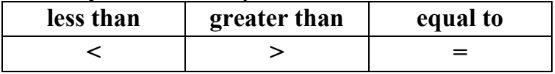• We can compare using number lines: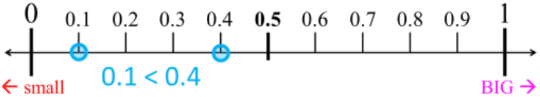• We can also compare using base ten (block) models: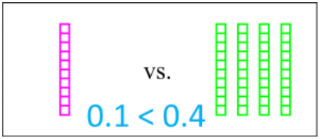• The steps for comparing two numbers:
2. Is the digit in this place value different between the two numbers?
1. YES: compare the digit (0 = smallest, 9 = biggest).
2. NO: move to the next smaller place value (right) and repeat step 2.
3. If all digits are the same in both numbers, they are equal.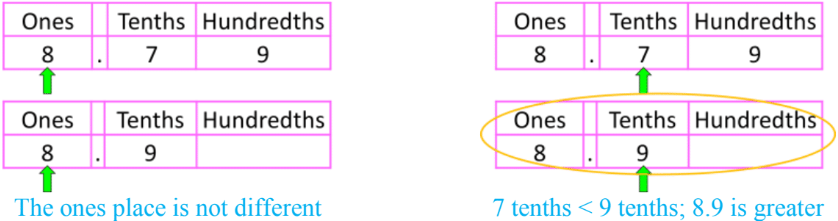• Once you have found a difference for a place value, it does not matter what numbers are in the places further on the right (smaller places)
• i.e. recall equivalent decimal tenths and hundredths 0.8=0.80
• ex. 8.9 > 8.79 (more decimal place values does not necessarily make the value greater)

• There are two ways to order a list of decimals:
• Ascending order: which means from least to greatest
• increasing; smallest to biggest (ex. 1.1 < 1.2 < 1.3 < 1.4)
• Descending order: which means from greatest to least
• decreasing; biggest to smallest (ex. 1.4 > 1.3 > 1.2 > 1.1)